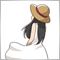# SnakeForce.mq4874

```void Drawing( int Shift)
{
double val,Dval;
val = 5 * (Mart[Shift] - Mart[ ArrayMinimum (Mart,cPeriod,Shift)]) / 9 ;
Dval = 5 * (Mart[Shift] -
Mart[Shift + 1 ] +
Mart[ ArrayMinimum (Mart,cPeriod,Shift + 1 )] -
Mart[ ArrayMinimum (Mart,cPeriod,Shift)]   ) / 9 ;
if (Dval > 0 )
{
ForceUp[Shift] = val;
ResistanceUp[Shift] = 0 ;
}
else
{
ForceUp[Shift] = 0 ;
ResistanceUp[Shift] = val;
}
val = 5 * (Mart[Shift] - Mart[ ArrayMaximum (Mart,cPeriod,Shift)]) / 9 ;
Dval = 5 * (Mart[Shift] -
Mart[Shift + 1 ] +
Mart[ ArrayMaximum (Mart,cPeriod,Shift + 1 )] -
Mart[ ArrayMaximum (Mart,cPeriod,Shift)]   ) / 9 ;
if (Dval < 0 )
{
ForceDown[Shift] = val;
ResistanceDown[Shift] = 0 ;
}
else
{
ForceDown[Shift] = 0 ;
ResistanceDown[Shift] = val;
}
return ;
}```

This is the Drawing function of SnakeForce.mq4.

After calculating the variable "Mart", it calculate ForceUp, ForceDown, ResistanceUp, ResistanceDown in this function, but I don't understand what do the numbers 5 and 9 mean here.

I know that this indicator repaints. I just want to know how to calculate Force and Resistance.25333

 Naguisa Unada: what do the numbers 5 and 9 mean here. It means 5/9 (0.5556) of the value in between. Nothing more.874

whroeder1 :
 It means 5/9 (0.5556) of the value in between. Nothing more.

Oh, is that something like that?

Thank you very much.